# Multiplicatively separable function

## Definition

### For a function of two variables

Suppose$G$ is a function of two variables$x$ and$y$. We say that$G$ is multiplicatively separable if there exist functions$f,g$ of one variable such that:$G(x,y) = f(x)g(y)$

on the entire domain of$G$.

Note that the concept of multiplicatively separable is sensitive to the coordinate system, i.e., if we change the coordinate system, a function that was originally multiplicatively separable need not remain multiplicatively separable.

### For a function of many variables

Suppose$G$ is a function of$n$ variables$x_1,x_2,\dots,x_n$. We say that$G$ is completely multiplicatively separable if there exist functions$g_1,g_2,\dots,g_n$, each a function of one variable, such that:$G(x_1,x_2,\dots,x_n) = g_1(x_1)g_2(x_2) \dots g_n(x_n)$

(note that the subscripts here are not to be confused with subscripts used for partial derivatives).

There is a weaker notion of partially multiplicatively separable: if we express the set$\{ 1,2,\dots,n\}$ as a union of two disjoint subsets$A,B$,$G$ is multiplicatively separable with respect to the partition if there exist functions$g_A,g_B$ such that: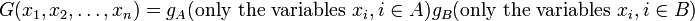$G(x_1,x_2,\dots,x_n) = g_A(\mbox{only the variables } x_i, i \in A)g_B(\mbox{only the variables } x_i, i \in B)$

## Partial derivatives

### For a function of two variables

Consider the case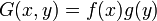$\! G(x,y) = f(x)g(y)$.

Then, if$f$ is$m$ times differentiable and$g$ is$n$ times differentiable, then$G_{xx\dots xyy\dots y}$ makes sense where$x$ occurs$m$ times and$y$ occurs$n$ times, and: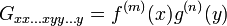$\! G_{xx\dots xyy\dots y} = f^{(m)}(x)g^{(n)}(y)$

Further, any partial derivative of$G$ that uses$m$ occurrences of$x$ and$n$ occurrences of$y$ will have the same derivative as the above.

In particular, we have that:

•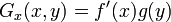$G_x(x,y) = f'(x)g(y)$
•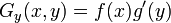$G_y(x,y) = f(x)g'(y)$
•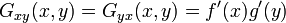$G_{xy}(x,y) = G_{yx}(x,y) = f'(x)g'(y)$

### For a function of many variables

Consider the case$G(x_1,x_2,\dots,x_n) = g_1(x_1)g_2(x_2) \dots g_n(x_n)$

Suppose$m_1,m_2,\dots,m_n$ are (possibly equal, possibly distinct) nonnegative integers such that each$f_i$ is$m_i$ times differentiable. Now, consider a partial derivative of$G$ that involves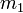$m_1$ differentiations in$x_1$,$m_2$ differentiations in$x_2$, and so on, with$m_i$ differentiations in each$x_i$. The order of the differentiations does not matter. Then, this partial derivative equals: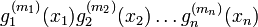$\! g_1^{(m_1)}(x_1)g_2^{(m_2)}(x_2) \dots g_n^{(m_n)}(x_n)$

## Integration on rectangular regions

### For a function of two variables

Suppose$G(x,y) = f(x)g(y)$ is a function of two variables. Consider a rectangular region$R$ of the form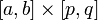$[a,b] \times [p,q]$ where$a,b,p,q$ are numbers. Then:$\int_R G(x,y) \, dA = \left(\int_a^b f(x) \, dx\right) \left(\int_p^q g(y) \, dy \right)$

### For a function of many variables

Suppose$G(x_1,x_2,\dots,x_n) = g_1(x_1)g_2(x_2) \dots g_n(x_n)$ is a function of$n$ variables. Consider a rectangular region$R$ of the form$[a_1,b_1] \times [a_2,b_2] \times \dots \times [a_n,b_n]$. Then: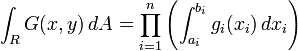$\int_R G(x,y) \, dA = \prod_{i=1}^n \left(\int_{a_i}^{b_i} g_i(x_i) \, dx_i \right)$